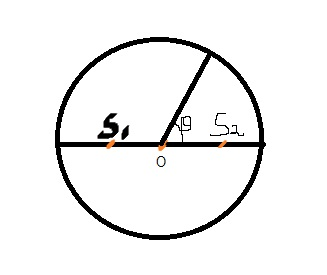# Wave Optics

Two coherent sources $S_{1}~and~S_{2}$ separated by distance $2 \lambda$ emit light of wavelength $\lambda$, in phase as shown. A circular wire of radius $100 \lambda$ is placed in such a way that $S_{1}S_{2}$ lies in its plane and the mid point of $S_{1}S_{2}$ is at the centre of wire. The angular positions on the wire for which intensity reduces to half of its maximum value for the first time is given as $\theta$. Find the value of $32cos \theta$×